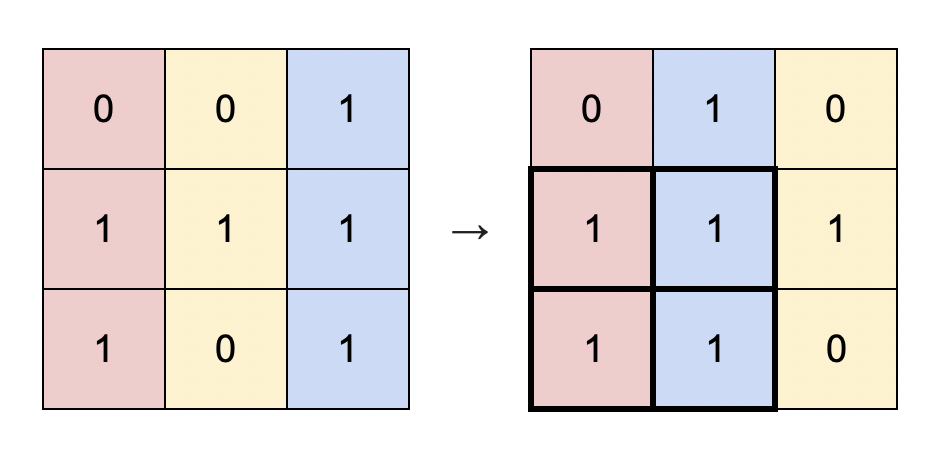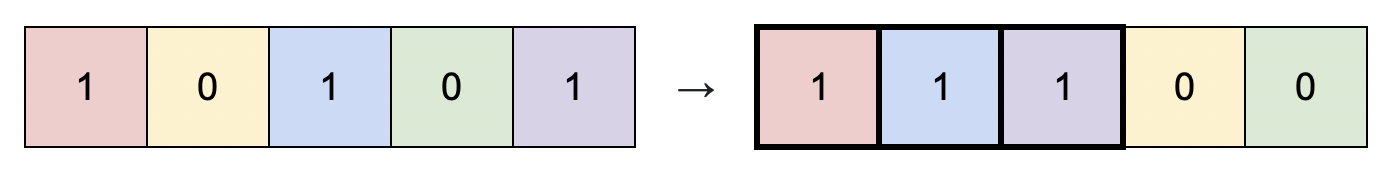Formatted question description: https://leetcode.ca/all/1727.html

# 1727. Largest Submatrix With Rearrangements

Medium

## Description

You are given a binary matrix matrix of size m x n, and you are allowed to rearrange the columns of the matrix in any order.

Return the area of the largest submatrix within matrix where every element of the submatrix is 1 after reordering the columns optimally.

Example 1:Input: matrix = [[0,0,1],[1,1,1],[1,0,1]]

Output: 4

Explanation: You can rearrange the columns as shown above.

The largest submatrix of 1s, in bold, has an area of 4.

Example 2:Input: matrix = [[1,0,1,0,1]]

Output: 3

Explanation: You can rearrange the columns as shown above.

The largest submatrix of 1s, in bold, has an area of 3.

Example 3:

Input: matrix = [[1,1,0],[1,0,1]]

Output: 2

Explanation: Notice that you must rearrange entire columns, and there is no way to make a submatrix of 1s larger than an area of 2.

Example 4:

Input: matrix = [[0,0],[0,0]]

Output: 0

Explanation: As there are no 1s, no submatrix of 1s can be formed and the area is 0.

Constraints:

• m == matrix.length
• n == matrix[i].length
• 1 <= m * n <= 10^5
• matrix[i][j] is 0 or 1.

## Solution

For each element in matrix, calculate the number of consecutive 1’s above the element. Concretely, if matrix[i][j] == 1, then find the minimum k such that for all k <= p <= i, there is matrix[p][j] == 1. Create newMatrix with the same size as matrix, and set newMatrix[i][j] = i - k + 1 where k is defined above.

Then for each row curRow in newMatrix, sort curRow and loop over curRow backwards. For curRow[j], the current area is calculated as (n - j) * curRow[j]. Maintain the maximum area in the process.

Finally, return the maximum area.

class Solution {
public int largestSubmatrix(int[][] matrix) {
int maxArea = 0;
int rows = matrix.length, columns = matrix.length;
int[][] newMatrix = new int[rows][columns];
for (int j = 0; j < columns; j++)
newMatrix[j] = matrix[j];
for (int i = 1; i < rows; i++) {
for (int j = 0; j < columns; j++) {
if (matrix[i][j] == 1)
newMatrix[i][j] = newMatrix[i - 1][j] + 1;
}
}
for (int i = 0; i < rows; i++) {
int[] curRow = new int[columns];
System.arraycopy(newMatrix[i], 0, curRow, 0, columns);
Arrays.sort(curRow);
for (int j = columns - 1; j >= 0; j--) {
if (curRow[j] == 0)
break;
int area = (columns - j) * curRow[j];
maxArea = Math.max(maxArea, area);
}
}
return maxArea;
}
}